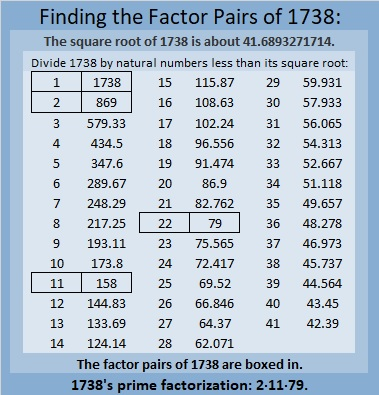# 1738 Little Surprises Around Every Turn

Contents

### Today’s Puzzle:

Is this mystery-level puzzle easy or difficult? I’m not saying. Place all the numbers from 1 to 12 in both the first column and the top row so that those numbers are the factors of the given clues. If you use logic to solve the puzzle, you will probably find some surprises around every turn.### Factors of 1738:

It may surprise you that 1738 is divisible by 11. Why is it? Because
1 – 7 + 3 – 8 = -11, a multiple of 11, which makes 1738 divisible by 11.

• 1738 is a composite number.
• Prime factorization: 1738 = 2 × 11 × 79.
• 1738 has no exponents greater than 1 in its prime factorization, so √1738 cannot be simplified.
• The exponents in the prime factorization are 1, 1, and 1. Adding one to each exponent and multiplying we get (1 + 1)(1 + 1)(1 + 1) = 2 × 2 × 2 = 8. Therefore 1738 has exactly 8 factors.
• The factors of 1738 are outlined with their factor pair partners in the graphic below.### More About the Number 1738:

Maybe you will be surprised by some of these number facts about the number 1738.

As mentioned in the table, 1738 = 2 × 11 × 79. That means 1738 is the short leg in the Pythagorean triple calculated from 2(79)(11), 79² – 11², 79² + 11².

1738 is the sum of eleven consecutive numbers:
153 + 154 + 155 + 156 +157 + 158 + 159 + 160 + 161 + 162 + 163 = 1738.

1738 is the sum of four consecutive numbers:
433 + 434 + 435 + 436 = 1738.

And because four is an even number:
436² – 435² + 434² – 433² = 1738.
Surprised?

1738 is also the sum of the 44 consecutive numbers from 18 to 61.

Consequently,
61² – 60² + 59² – 68² + 57² – 56² + 55² – 54² + 53² – 52² + 51² – 50² + 49² – 48² + 47² – 46² + 45² – 44² + 43² – 42² + 41² – 40² + 39² – 38² + 37² – 36² + 35² – 34² + 33² – 32² + 31² – 30² + 29² – 28² + 27² – 26² + 25² – 24² + 23² – 22² + 21² – 20² + 19² – 18² = 1738. I bet you weren’t expecting that!

But the biggest surprise about 1738 is something I learned from OEIS.org: 1738 is in an equation that uses every digit from 1 to 9 exactly one time:make science GIFs like this at MakeaGif

This site uses Akismet to reduce spam. Learn how your comment data is processed.